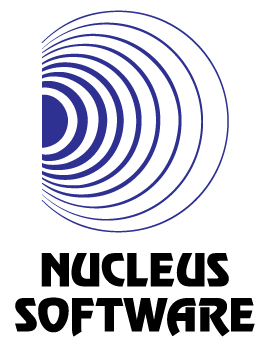Nanachi and Euler Totient
Tag(s):

## Algebra, Easy, Euler's totient function, Mathematics, Number Theory

Problem
Editorial
Analytics

Living alone with Mitty in the Abyss, Nanachi has developed an interest in number theory problems. Nanachi recently came up with the following problem but is unable to solve it.

Let $\phi(n)$ denote the count of positive integers up to n which are coprime with n. Since summations bore our Nanachi, Nanachi decided to evaluate the function $f(n)$ instead which is defined as:

$\displaystyle f(n) = \prod_{d|n} \phi(d)$

where $a|b$ mean a divides b. Nanachi seeks your help in finding the value of $f(n)$.

INPUT
A single integer n

OUTPUT
Value of $f(n)$. Since this value can be large, output it modulo $10^9 + 7$.

CONSTRAINTS
$1 \leq n \leq 10^{12}$

For 10% of the testcases, $n \leq 10^6$

SAMPLE INPUT
12

SAMPLE OUTPUT
32

Explanation

The divisors of $12$ are $1, 2, 3, 4, 6$ and $12$ i.e $f(n) = \phi(1)\cdot\phi(2)\cdot\phi(3)\cdot\phi(4)\cdot\phi(6)\cdot \phi(12)$

$\Rightarrow f(n) = 1\cdot 1\cdot 2\cdot 2\cdot 2\cdot 4 = 32$

Time Limit: 1.0 sec(s) for each input file.
Memory Limit: 256 MB
Source Limit: 1024 KB
Marking Scheme: Marks are awarded when all the testcases pass.
Allowed Languages: Bash, C, C++, C++14, Clojure, C#, D, Erlang, F#, Go, Groovy, Haskell, Java, Java 8, JavaScript(Rhino), JavaScript(Node.js), Julia, Kotlin, Lisp, Lisp (SBCL), Lua, Objective-C, OCaml, Octave, Pascal, Perl, PHP, Python, Python 3, R(RScript), Racket, Ruby, Rust, Scala, Swift, Swift-4.1, TypeScript, Visual Basic

## CODE EDITOR

Initializing Code Editor...

## This Problem was Asked inChallenge Name

Nucleus Software Coding Challenge

OTHER PROBLEMS OF THIS CHALLENGE
• Algorithms > Searching
• Algorithms > Dynamic Programming